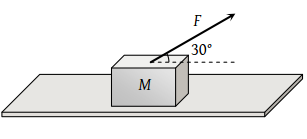On the horizontal surface of a truck (μ = 0.6), a block of mass 1 kg is placed. If the truck is accelerating at the rate of 5m/sec2 then frictional force on the block will be

(1) 5 N

(2) 6 N

(3) 5.88 N

(4) 8 N

Concept Questions :-

Pseudo force
High Yielding Test Series + Question Bank - NEET 2020

Difficulty Level:

A vehicle of mass m is moving on a rough horizontal road with momentum P. If the coefficient of friction between the tyres and the road be μ, then the stopping distance is

(1) $\frac{P}{2\mu \text{\hspace{0.17em}}m\text{\hspace{0.17em}}g}$

(2) $\frac{{P}^{2}}{2\mu \text{\hspace{0.17em}}m\text{\hspace{0.17em}}g}$

(3) $\frac{P}{2\mu \text{\hspace{0.17em}}m{\text{\hspace{0.17em}}}^{2}g}$

(4) $\frac{{P}^{2}}{2\mu \text{\hspace{0.17em}}m{\text{\hspace{0.17em}}}^{2}g}$

Concept Questions :-

Friction
High Yielding Test Series + Question Bank - NEET 2020

Difficulty Level:

A block of mass M = 5 kg is resting on a rough horizontal surface for which the coefficient of friction is 0.2. When a force F = 40 N is applied, the acceleration of the block will be (g = 10 m/s2)(1) 5.73 m/sec2

(2) 8.0 m/sec2

(3) 3.17 m/sec2

(4) 10.0 m/$se{c}^{2}$

Concept Questions :-

Friction
High Yielding Test Series + Question Bank - NEET 2020

Difficulty Level:

Consider a car moving on a straight road with a speed of 100 m/s. The distance at which car can be stopped is $\left[{\mu }_{k}=0.5\right]$

(1) 100 m

(2) 400 m

(3) 800 m

(4) 1000 m

Concept Questions :-

Friction
High Yielding Test Series + Question Bank - NEET 2020

Difficulty Level:

A given object takes n times as much time to slide down a 45° rough incline as it takes to slide down a perfectly smooth 45° incline. The coefficient of kinetic friction between the object and the incline is given by

(1) $\left(1-\frac{1}{{n}^{2}}\right)$

(2) $\frac{1}{1-{n}^{2}}$

(3) $\sqrt{\left(1-\frac{1}{{n}^{2}}\right)}$

(4) $\sqrt{\frac{1}{1-{n}^{2}}}$

Concept Questions :-

Friction
High Yielding Test Series + Question Bank - NEET 2020

Difficulty Level:

Starting from rest, a body slides down a 45° inclined plane in twice the time it takes to slide down the same distance in the absence of friction. The coefficient of friction between the body and the inclined plane is

(1) 0.33

(2) 0.25

(3) 0.75

(4) 0.80

Concept Questions :-

Friction
High Yielding Test Series + Question Bank - NEET 2020

Difficulty Level:

A force of 750 N is applied to a block of mass 102 kg to prevent it from sliding on a plane with an inclination angle 30° with the horizontal. If the coefficients of static friction and kinetic friction between the block and the plane are 0.4 and 0.3 respectively, then the frictional force acting on the block is

(1) 750 N

(2) 500 N

(3) 345 N

(4) 250 N

Concept Questions :-

Friction
High Yielding Test Series + Question Bank - NEET 2020

Difficulty Level:

A body takes just twice the time as long to slide down a plane inclined at 30o to the horizontal as if the plane were frictionless. The coefficient of friction between the body and the plane is

(1) $\frac{\sqrt{3}}{4}$

(2) $\sqrt{3}$

(3) $\frac{4}{3}$

(4) $\frac{3}{4}$

Concept Questions :-

Friction
High Yielding Test Series + Question Bank - NEET 2020

Difficulty Level:

A body takes time t to reach the bottom of an inclined plane of angle θ with the horizontal. If the plane is made rough, time taken now is 2t. The coefficient of friction of the rough surface is

(1) $\frac{3}{4}\mathrm{tan}\theta$

(2) $\frac{2}{3}\mathrm{tan}\theta$

(3) $\frac{1}{4}\mathrm{tan}\theta$

(4) $\frac{1}{2}\mathrm{tan}\theta$

Concept Questions :-

Friction
High Yielding Test Series + Question Bank - NEET 2020

Difficulty Level:

A block is kept on an inclined plane of inclination θ of length l. The velocity of particle at the bottom of inclined is (the coefficient of friction is μ)

(1) $\sqrt{2gl\left(\mu \mathrm{cos}\theta -\mathrm{sin}\theta \right)}$

(2) $\sqrt{2gl\left(\mathrm{sin}\theta -\mu \mathrm{cos}\theta \right)}$

(3) $\sqrt{2gl\left(\mathrm{sin}\theta +\mu \mathrm{cos}\theta \right)}$

(4) $\sqrt{2gl\left(\mathrm{cos}\theta +\mu \mathrm{sin}\theta \right)}$

Concept Questions :-

Friction